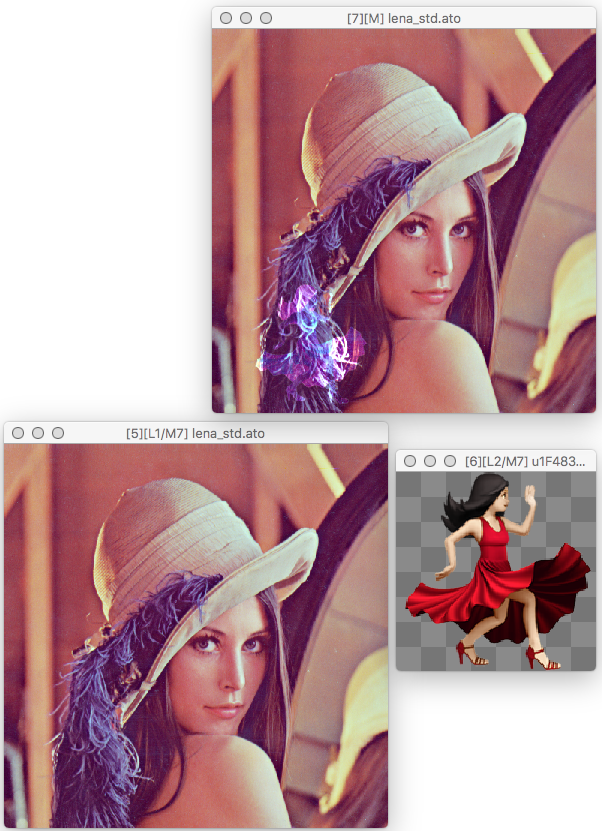Layers Dialog — .elx Files / Layer Blending Modes / Traditional Blend Modes

#### § 19.6.1.9 - Divide

This mode divides the content of the lower layers by the upper layer.

The channels are treated as a value of 2 where they are zero; 1.5 where they are 32768, and 1 where they are 65535. The result is unchanged where the upper layer is white; 1.5x intensity where the upper layer channels are all 32768 (1.5), and double intensity where the upper channels are zero (2.)

Where the higher layer is partially transparent, the content is mixed with the lower layer composite in a manner proportional to the amount of transparency.

Tip: Divide is not the exact opposite of Multiply mode; Divide can double the brightness of a layer, while Multiply can only bring the layer to normal brightness.Divide blend mode